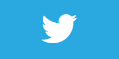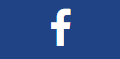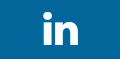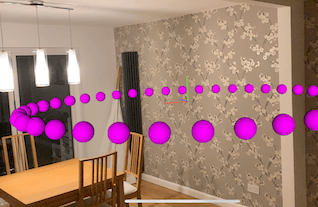# Place nodes at regular intervals## Description

If we want to place nodes an equal distance apart from a central point (for example the world origin), we can use some maths to determine the positions of those nodes as we would the cordinates of vertices of an n sided polygon with a known radius.

For example, if we had 3 items we wanted to position around the user they would be at the 3 points of a triangle, 4 points a square, 5 points a pentagon etc

What if there are 40 items you want to place around the user at regular intervals? Then we need to calculate the position of the vertices of a 40 sided regular polygon. As can be seen in the video and code below.

## Code

``````using ARKit;
using SceneKit;
using System;
using UIKit;

namespace XamarinArkitSample
{
public partial class ViewController : UIViewController
{

public ViewController(IntPtr handle) : base(handle)
{
this.sceneView = new ARSCNView
{
AutoenablesDefaultLighting = true,
DebugOptions = ARSCNDebugOptions.ShowFeaturePoints
| ARSCNDebugOptions.ShowWorldOrigin
};

}

{

this.sceneView.Frame = this.View.Frame;
}

public override void ViewDidAppear(bool animated)
{
base.ViewDidAppear(animated);

this.sceneView.Session.Run(new ARWorldTrackingConfiguration
{
AutoFocusEnabled = true,
PlaneDetection = ARPlaneDetection.Horizontal,
LightEstimationEnabled = true,
}, ARSessionRunOptions.ResetTracking | ARSessionRunOptions.RemoveExistingAnchors);

var radius = 1.2f; // 1.2m away from world origin

var sides = 40;

for (int i = 0; i < sides; i++)
{
float x = (float)(radius * Math.Cos(2 * Math.PI * i / sides));
var y = 0f;
var z = (float)(radius * Math.Sin(2 * Math.PI * i / sides));

var node = new SphereNode(0.05f, UIColor.Magenta);
node.Position = new SCNVector3(x, y, z);

}
}

public override void ViewDidDisappear(bool animated)
{
base.ViewDidDisappear(animated);

this.sceneView.Session.Pause();
}

{
}
}

public class SphereNode : SCNNode
{
public SphereNode(float size, UIColor color)
{
var rootNode = new SCNNode
{
Geometry = CreateGeometry(size, color),
};

}

private static SCNGeometry CreateGeometry(float size, UIColor color)
{
var material = new SCNMaterial();
material.Diffuse.Contents = color;

var geometry = SCNSphere.Create(size);
geometry.Materials = new[] { material };

return geometry;
}
}
}
``````

## Next Step : Use physics in scene

After you have mastered this you should try Use physics in scene